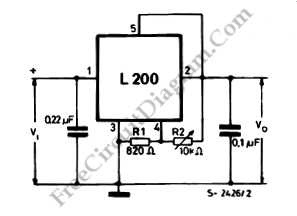## Low Drop-Out (LDO) Voltage Regulator Using Discrete Semiconductors

There are several integrated circuit chips designed specially for LDO (low drop out) voltage regulator. Building LDO using standard op-amp and discrete components can be an alternative. Here is one design of LDO circuit: Here some guidelines to select the components values: D1 is chosen for the best performance, the sharper the on-off transition curve the better. Some people say […]

## Synchronized Mains Voltage Power Control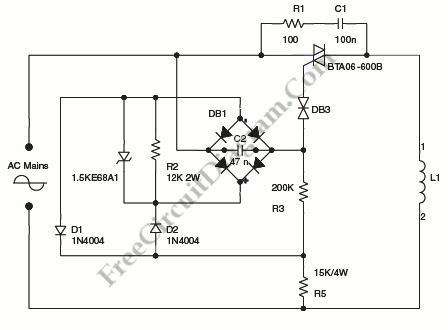A step-down circuit used to approximately divide by two the rms voltage between the line input and the inductive load (L1) is shown on circuit diagram below. A turn-on delay of approximately 7 ms is provided by C2 and R3. We can discharge C2 at each line zero voltage using diode bridge, with diodes D1 and D2 and R2 resistor. […]

## Precision Power Regulator with Low Temperature Coefficient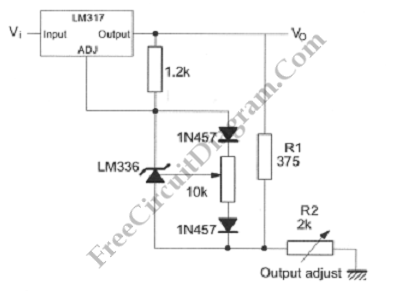Making a precision voltage source is easy, but not so easy if the voltage should be consistent over wide range of ambient temperature. Such requirement might be needed in high precision measurement system, for providing reference voltage in analog to digital conversion for example. Here is the circuit’s schematic diagram: The LM236, LM336 voltage references are easier to use than […]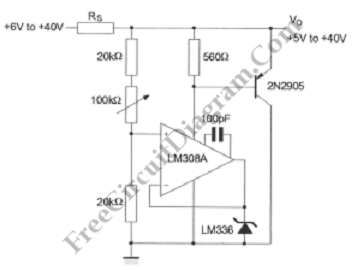This shunt regulator acts like a Zener diode, but we can adjust the voltage (via a potentiometer). Here is the schematic diagram of the circuit: When the maximum load current is known, then we can choose Rs such as that Rload (at max current) and Rs form a voltage divider that gives a voltage level at slightly higher than the […]

## Soft Start Mechanism for L200 Voltage Regulator

The configuration in the circuit diagram below can be used when a slow rise time of the output voltage is required. We can find the rise time with following formula: ton= CVoR 0.45 Capacitor C is discharged when the switch position is ON and this will maintain the voltage at pin 2 low. The Vo follows the voltage at pin […]

## Reducing L200 Power Dissipation using Series Resistor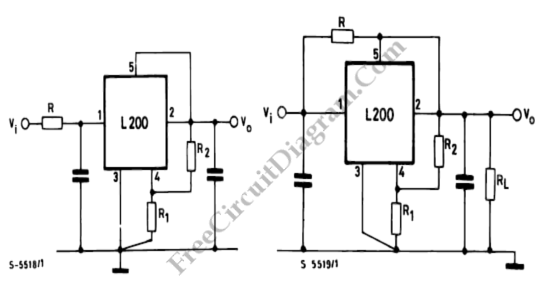It’s good to reduce the power dissipated by the device. Using resistor connected in series to the input (the left figure) is a simple and economic method to reduce the device input-output differential voltage. Here’s the formula for calculating R: R= [Vi min- (Vo+Vdrop)]/Io Vdrop = minimum differential voltage between the input and the output of the device at current […]